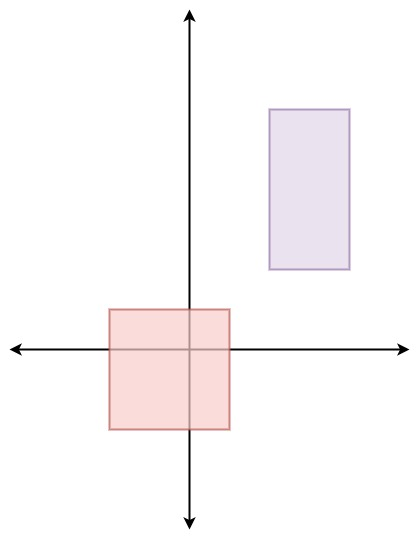497. Random Point in Non-overlapping Rectangles
Medium
421
639

You are given an array of non-overlapping axis-aligned rectangles `rects` where `rects[i] = [ai, bi, xi, yi]` indicates that `(ai, bi)` is the bottom-left corner point of the `ith` rectangle and `(xi, yi)` is the top-right corner point of the `ith` rectangle. Design an algorithm to pick a random integer point inside the space covered by one of the given rectangles. A point on the perimeter of a rectangle is included in the space covered by the rectangle.

Any integer point inside the space covered by one of the given rectangles should be equally likely to be returned.

Note that an integer point is a point that has integer coordinates.

Implement the `Solution` class:

• `Solution(int[][] rects)` Initializes the object with the given rectangles `rects`.
• `int[] pick()` Returns a random integer point `[u, v]` inside the space covered by one of the given rectangles.

Example 1:```Input
["Solution", "pick", "pick", "pick", "pick", "pick"]
[[[[-2, -2, 1, 1], [2, 2, 4, 6]]], [], [], [], [], []]
Output
[null, [1, -2], [1, -1], [-1, -2], [-2, -2], [0, 0]]

Explanation
Solution solution = new Solution([[-2, -2, 1, 1], [2, 2, 4, 6]]);
solution.pick(); // return [1, -2]
solution.pick(); // return [1, -1]
solution.pick(); // return [-1, -2]
solution.pick(); // return [-2, -2]
solution.pick(); // return [0, 0]
```

Constraints:

• `1 <= rects.length <= 100`
• `rects[i].length == 4`
• `-109 <= ai < xi <= 109`
• `-109 <= bi < yi <= 109`
• `xi - ai <= 2000`
• `yi - bi <= 2000`
• All the rectangles do not overlap.
• At most `104` calls will be made to `pick`.
Accepted
36.8K
Submissions
93.5K
Acceptance Rate
39.4%

Seen this question in a real interview before?
1/4
Yes
No

Discussion (0)

Related Topics